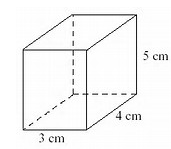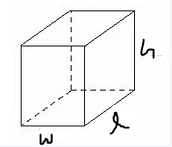Mathematics
Easy

Question

# Sam is using this box to pack books. He needs to know the volume so he can figure out how many books to pack. Write expression below would Sam use to find the volume of the box.## 3 + 4 + 53 + 4 × 53 × 4 + 53 × 4 × 5Hint:

## The correct answer is: 3 × 4 × 5

### V = l × w × h= 3 × 4 × 5Sam use to find the volume of the box is 3 × 4 × 5.

The dimensions are given as:The volume of the box is given by:

V = l x w x h

On comparing the images above, we can see that

l = 4 cm

w = 3 cm

h = 5 cm

Thus,

V = 4 x 3 x 5

The correct option is (d) 3 × 4 × 5

### Related Questions to study#### With Turito Foundation.#### Get an Expert Advice From Turito.